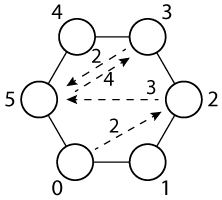English translationWe have a board with fields numbered from 0 to 5 (because computer scientists count from 0, not from 1). We start on 0 and roll a dice. Let us say, for example, that the first number is 2 (we move to 2), then 3 (we go to 5), then 4 (we go to 3 because the board is -- unlike the Earth - round), next is 2 (we are on 5 again) ...

Write a program that simulates such a game and prints out the number of moves necessary to reach the field 5 for 100 times.

We simulate the dice with

from random import *
seed(8)

After this, every call randint(1, 6) will return a random number between 1 and 6.

Program

print(randint(1, 6))
print(randint(1, 6))
print(randint(1, 6))
print(randint(1, 6))

prints (coincidentally :)

2
3
4
2

Because the dice will always return the same numbers (due to call seed(8) - the number 8 could also be any other number, resulting in a different sequence of random numbers), we actually know the correct results that should be printed out: 690.